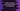# How to print keys and values of a python dictionary# Python print dictionary keys and values :

In this tutorial, we will learn how to print the keys and values of a dictionary in python. For printing the keys and values, we can either iterate through the dictionary one by one and print all key-value pairs or we can print all keys or values at one go. For this tutorial, we are using python 3.

This is the simplest way to print all key-value pairs of a dictionary. Using one for loop, we will iterate through each element of the dictionary_ one by one_ and then print them out. The code will look like as below :

``````my_dict = {"one": 1,"two":2,"three":3,"four":4}

for item in my_dict:
print("Key : {} , Value : {}".format(item,my_dict[item]))``````

Here, we are iterating through each element of the dictionary using a for loop. This program will print out the below output :

``````Key : one , Value : 1
Key : two , Value : 2
Key : three , Value : 3
Key : four , Value : 4``````

As you can see that all keys and values are printed out.

## Using items() method :

We can also use items() method to create one list from a dictionary and then we can iterate through all_ key-value_ pairs.

``````my_dict = {"one": 1,"two":2,"three":3,"four":4}

for key,value in my_dict.items():
print("Key : {} , Value : {}".format(key,value))``````

It will print the same output as the previous example.

## By iterating through all keys :

Python provides one _keys() _method to get all keys from a python dictionary. Then we can iterate through the keys one by one and print out the value for each key.

``````my_dict = {"one": 1,"two":2,"three":3,"four":4}

for key in my_dict.keys():
print("Key : {} , Value : {}".format(key,my_dict[key]))``````

This program will print the same output as the above two.

### Conclusion :

You can use any of the above three methods to iterate through all_ key-value_ pairs of a python dictionary. Try to run the above programs on your PC and drop one comment below if you have any queries.